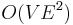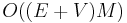# Edmonds–Karp algorithm

The Edmonds–Karp algorithm is a special case of the Ford–Fulkerson method that always chooses a shortest augmenting path at each iteration. It solves the maximum flow problem in$O(VE^2)$ time.

## The algorithm

```input flow network (V, s, t, c)
F ← 0
for u ∈ V
for v ∈ V
f[u, v] ← 0
r[u, v] ← c[u, v]
while t is reachable from s in (V, s, t, r)
find a shortest augmenting path (s = v, v, ..., v[n] = t) with BFS
m ← ∞
for i ∈ [0, n)
m ← min(m, r[v[i], v[i+1]])
for i ∈ [0, n)
f[v[i], v[i+1]] ← f[v[i], v[i+1]] + m
f[v[i+1], v[i]] ← f[v[i+1], v[i]] - m
r[v[i], v[i+1]] ← r[v[i], v[i+1]] - m
r[v[i+1], v[i]] ← r[v[i+1], v[i]] + m
F ← F + m
output max flow f
output max flow value F
```

## Complexity

A trivial upper bound of$O((E+V)M)$ can be derived in the case that all edge capacities are integers. Each iteration of the algorithm uses breadth-first search, which takes$O(E+V)$ time, to find a shortest augmenting path, and there are at most$M$ iterations, where$M$ is the value of the max flow, since in each step the total flow so far increases by at least one unit.

As a matter of fact, however, the Edmonds–Karp algorithm's running time has a capacity-independent bound,$O(VE^2)$. The proof is not trivial and can be found in a textbook such as Introduction to Algorithms by Cormen et al.